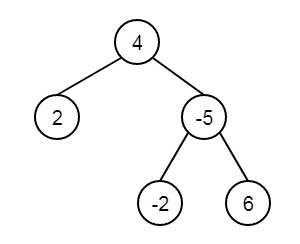# Find the closest leaf in a Binary Tree in C++

Suppose, one binary tree is given. It has leaf nodes at different levels. Another pointer is given, that is pointing to a node. We have to find the distance to the nearest leaf node from the pointed node. Consider the tree is like below −Here leaf nodes are 2, -2 and 6. If the pointer is pointing to node -5, The nearest nodes from -5 will be at distance 1.

To solve this, we will traverse the subtree rooted with the given node, and find the closest leaf in the subtree, then store the distance. Now traversing tree starting from the root, if the node x is present in the left subtree, then search into the right subtree, and vice versa.

## Example

Live Demo

#include<iostream>
using namespace std;
class Node {
public:
int data;
Node *left, *right;
};
Node* getNode(int data) {
Node* node = new Node;
node->data = data;
node->left = node->right = NULL;
return node;
}
void getLeafDownward(Node *root, int level, int *minDist) {
if (root == NULL)
return ;
if (root->left == NULL && root->right == NULL) {
if (level < (*minDist))
*minDist = level;
return;
}
getLeafDownward(root->left, level+1, minDist);
getLeafDownward(root->right, level+1, minDist);
}
int getFromParent(Node * root, Node *x, int *minDist) {
if (root == NULL)
return -1;
if (root == x)
return 0;
int l = getFromParent(root->left, x, minDist);
if (l != -1) {
getLeafDownward(root->right, l+2, minDist);
return l+1;
}
int r = getFromParent(root->right, x, minDist);
if (r != -1) {
getLeafDownward(root->left, r+2, minDist);
return r+1;
}
return -1;
}
int minimumDistance(Node *root, Node *x) {
int minDist = INT8_MAX;
getLeafDownward(x, 0, &minDist);
getFromParent(root, x, &minDist);
return minDist;
}
int main() {
Node* root = getNode(4);
root->left = getNode(2);
root->right = getNode(-5);
root->right->left = getNode(-2);
root->right->right = getNode(6);
Node *x = root->right;
cout << "Closest distance of leaf from " << x->data <<" is: " << minimumDistance(root, x);
}

## Output

Closest distance of leaf from -5 is: 1